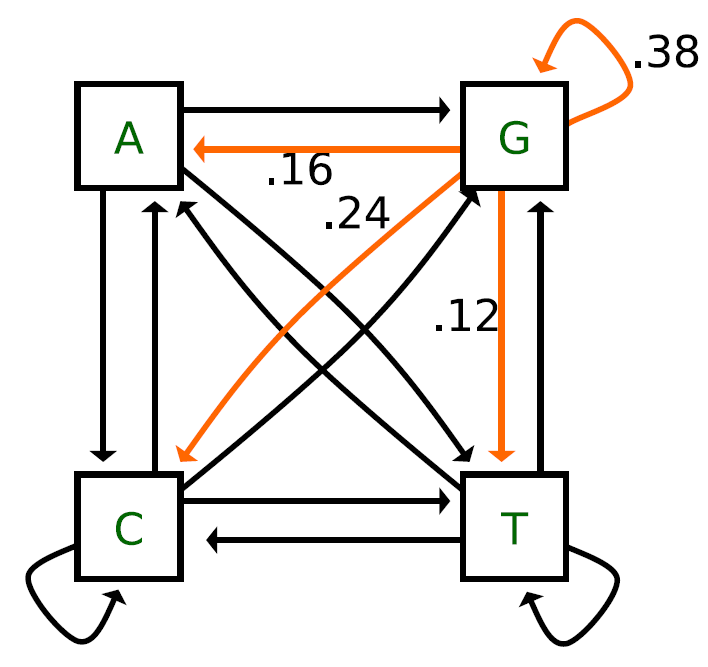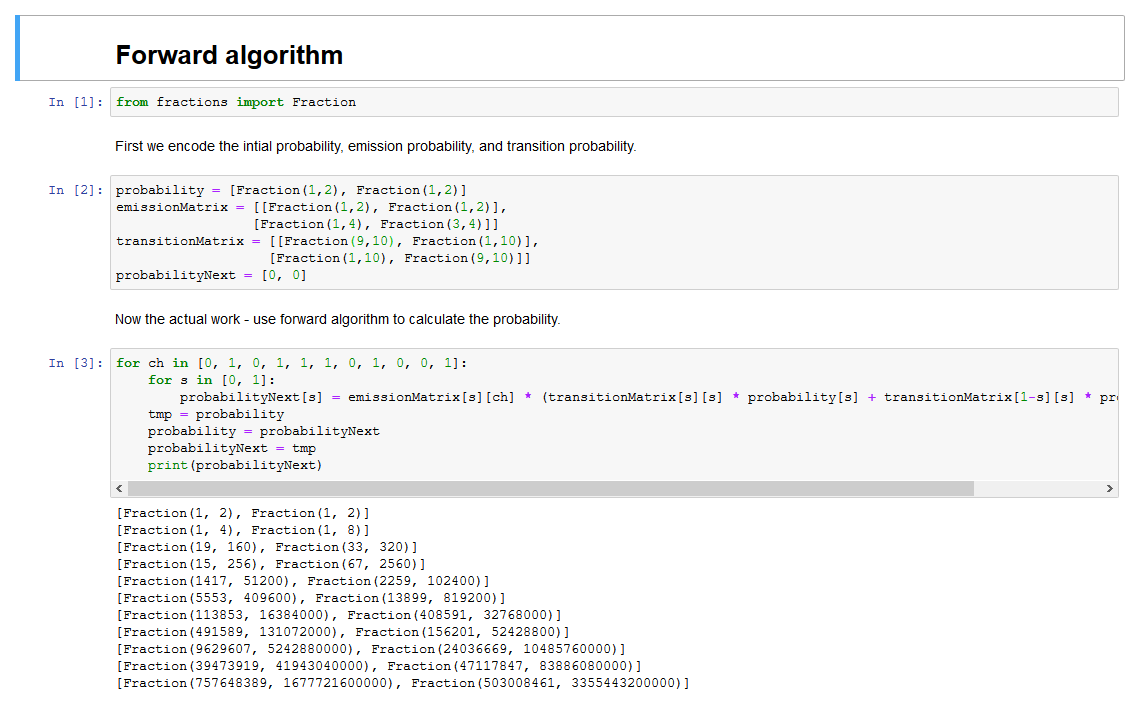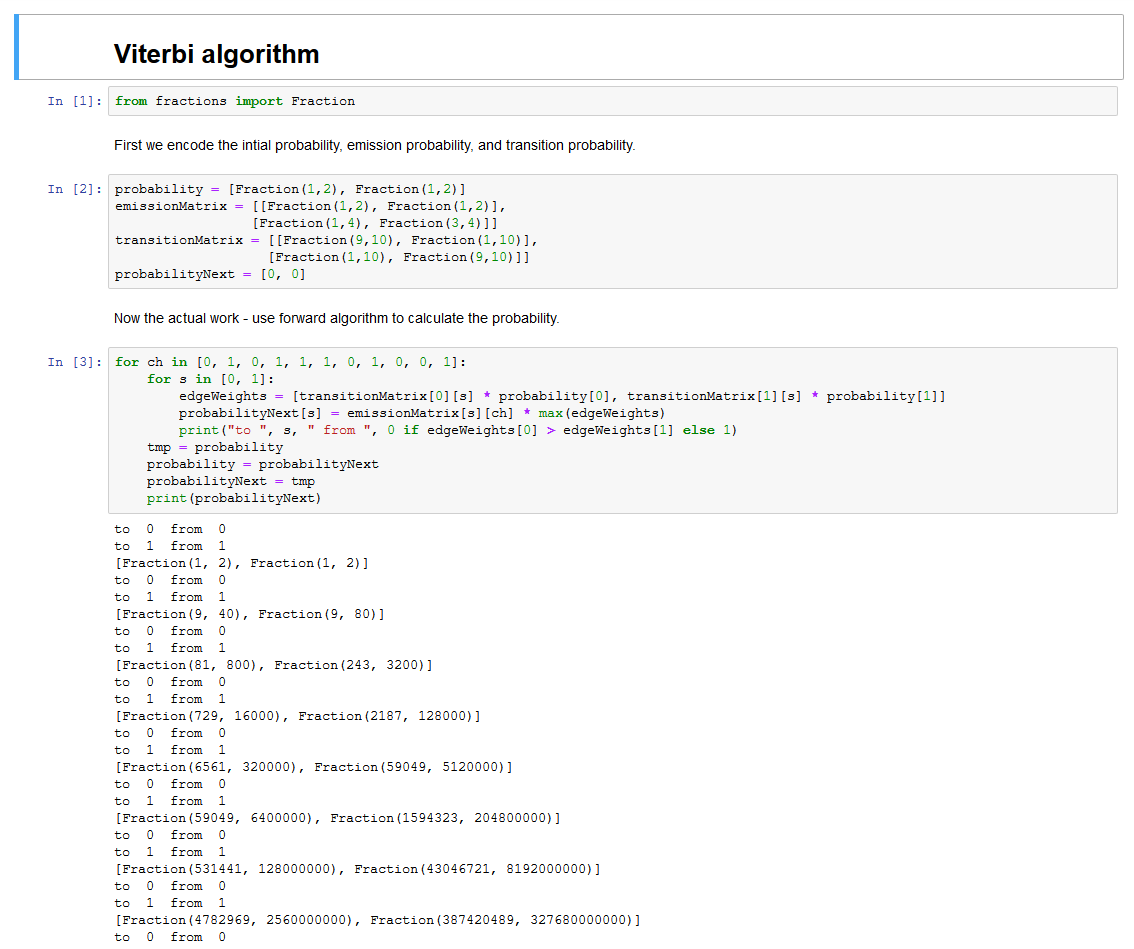Warning

# Tutorial 7 - Hidden Markov models

In this tutorial, we will study Markov models. Markov model is a four-tuple $(\Sigma, S, P_t, P_0)$ where

• $\Sigma$ is an alphabet,
• $S$ is a set of states,
• $P_t : S \times S \mapsto [0,1]$ is a matrix of transition probabilities, and
• $P_0 : S \mapsto [0, 1]$ is initial probability distribution on the states.

The Markovian property is useful as for any sequence $x \in \Sigma^*$ $$P(x) = P_0(x_1) \cdot P_t(x_2 \mid x_1) \cdot P_t(x_3 \mid x_2) \cdots P_t(x_l \mid x_{l-1}).$$

Consider the following Markov model:How would you calculate a probability of a sequence?

Is there anything missing?

How long can be sequences generated by this model?

Hidden Markov models separate states from the observable symbols. Each state generates a symbol that is visible to us. To modify the model, we have to add emission probabilities:

• $P_e : S \times \Sigma \mapsto [0,1]$

## Problem 1 - design an HMM diagram

Imagine that you are in a casino playing a game. The dealer is tossing a coin, and you bet the outcome. However, a friend notified you in advance that the dealer is a crook and sometimes he replaces the fair coin with a biased one and then the game follows with the biased coin for several moves. The biased coin generates heads with probability $\frac{3}{4}$, but the coins are visually indistinguishable. The dealer is cautious and replaces the coin only in one of ten tosses on average. Describe such a situation as a hidden Markov model, draw a diagram, write the set of states and set of observed symbols and show the transition probabilities in the diagram.

## Problem 2 - calculate the probability of a sequence

Using the model developed in the last example, calculate the probability that the sequence of states $\pi = \mathrm{FFFBBBBBFFF}$ generated sequence $01011101001$. Assume that the initial probabilities of fair and biased coins are equal.

## Problem 3 - Forward algorithm

In the last problem, we assumed that we know the sequence of hidden states. This is, however, usually not true. There are two possible solutions. First, we may try to enumerate all possible paths $\pi$, which is untractable. The second option is to use dynamic programming which leads to the forward algorithm. Use the forward algorithm to calculate the probability of sequence $01011101001$.

The problem is hard to calculate by hand. Calculate the first few symbols and then use either jupyter notebook from this archive or the python code below.

2020: If you want to get a better practice, try to code the forward algorithm yourself.

python

from fractions import Fraction

probability = [Fraction(1,2), Fraction(1,2)]
emissionMatrix = [[Fraction(1,2), Fraction(1,2)],
[Fraction(1,4), Fraction(3,4)]]
transitionMatrix = [[Fraction(9,10), Fraction(1,10)],
[Fraction(1,10), Fraction(9,10)]]
probabilityNext = [0, 0]

for ch in [0, 1, 0, 1, 1, 1, 0, 1, 0, 0, 1]:
for s in [0, 1]:
probabilityNext[s] = emissionMatrix[s][ch] * (transitionMatrix[s][s] * probability[s] + transitionMatrix[1-s][s] * probability[1-s])
tmp = probability
probability = probabilityNext
probabilityNext = tmp
print(probabilityNext)## Problem 4 - Viterbi algorithm

We already know how to calculate the probability of a sequence of observations. Another very useful case may be to calculate the most likely sequence of hidden states. This can be done by the Viterbi algorithm. Calculate the most likely sequence of states for the observed sequence $01011101001$.

The problem is hard to calculate by hand. Calculate the first few symbols and then use either jupyter notebook from this archive or the python code below.

python

from fractions import Fraction

probability = [Fraction(1,2), Fraction(1,2)]
emissionMatrix = [[Fraction(1,2), Fraction(1,2)],
[Fraction(1,4), Fraction(3,4)]]
transitionMatrix = [[Fraction(9,10), Fraction(1,10)],
[Fraction(1,10), Fraction(9,10)]]
probabilityNext = [0, 0]

for ch in [0, 1, 0, 1, 1, 1, 0, 1, 0, 0, 1]:
for s in [0, 1]:
edgeWeights = [transitionMatrix[s] * probability, transitionMatrix[s] * probability]
probabilityNext[s] = emissionMatrix[s][ch] * max(edgeWeights)
print("to ", s, " from ", 0 if edgeWeights > edgeWeights else 1)
tmp = probability
probability = probabilityNext
probabilityNext = tmp
print(probabilityNext)## Assignment 3 - implement $\mathrm{CpG}$-island classifier

In the problems, we considered only a simple model with a fair and a biased coin. However, this approach applies to bioinformatics. Instead of heads and tails, we may work with nucleotides. The hidden states may represent genes or, as in the assignment, $\mathrm{CpG}$-islands.

Work individually on the third programming assignment. The deadline is on April 21, 2019. Upload in BRUTE.

This tutorial is adapted from chapter 11 from the book Introduction to bioinformatics algorithms by N. Jones and P. Pevzner. https://mitpress.mit.edu/books/introduction-bioinformatics-algorithms.

For further explanation, you may visit slides from the past years.